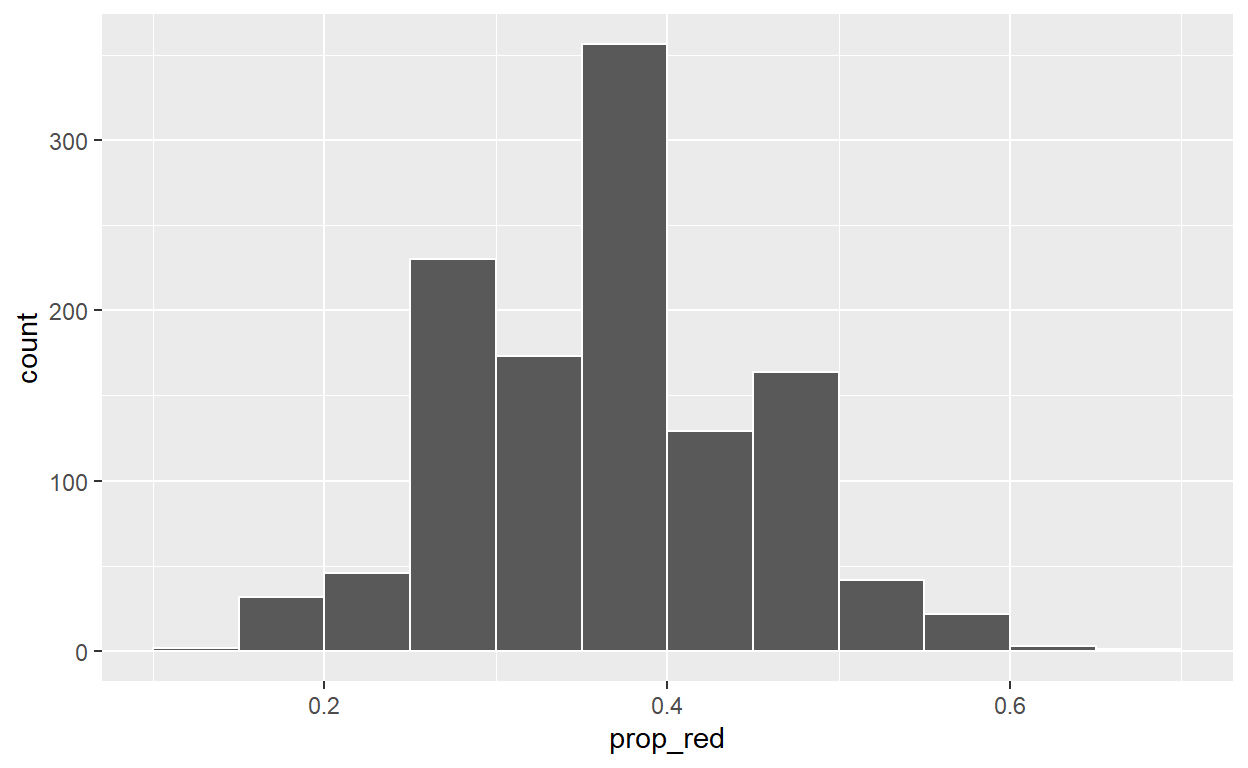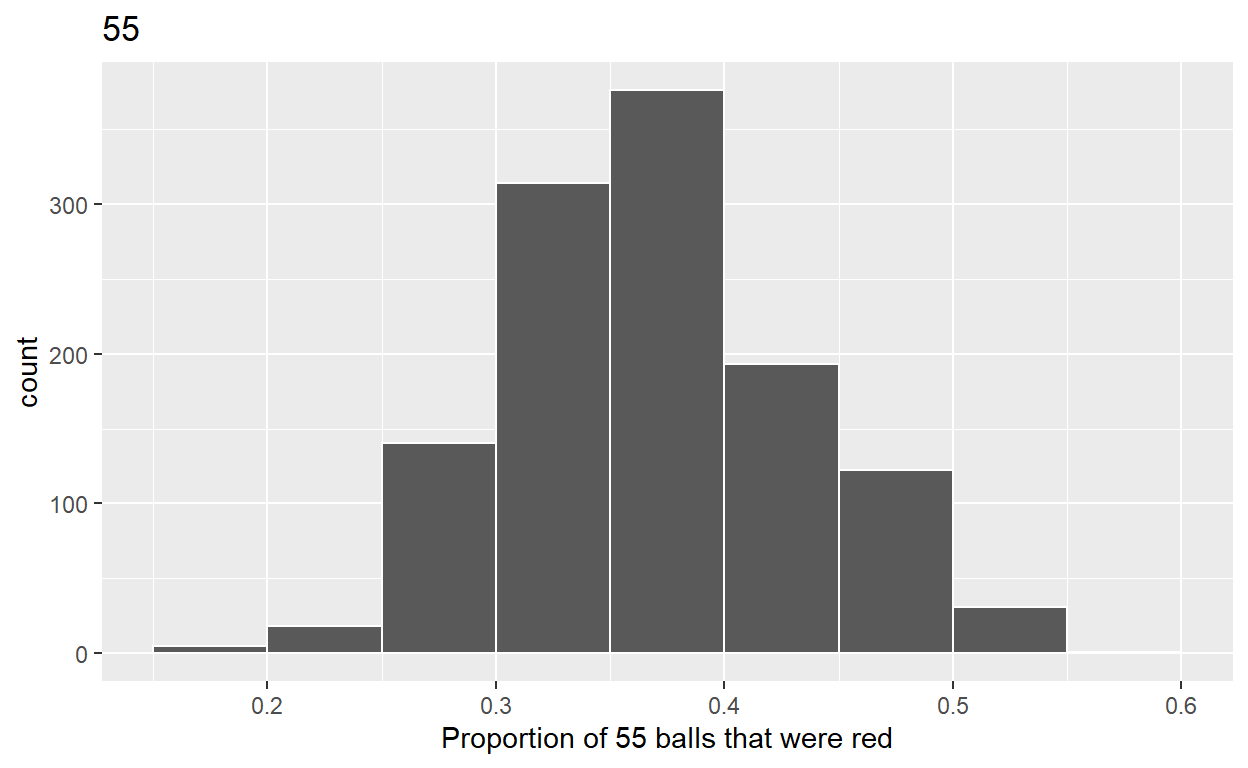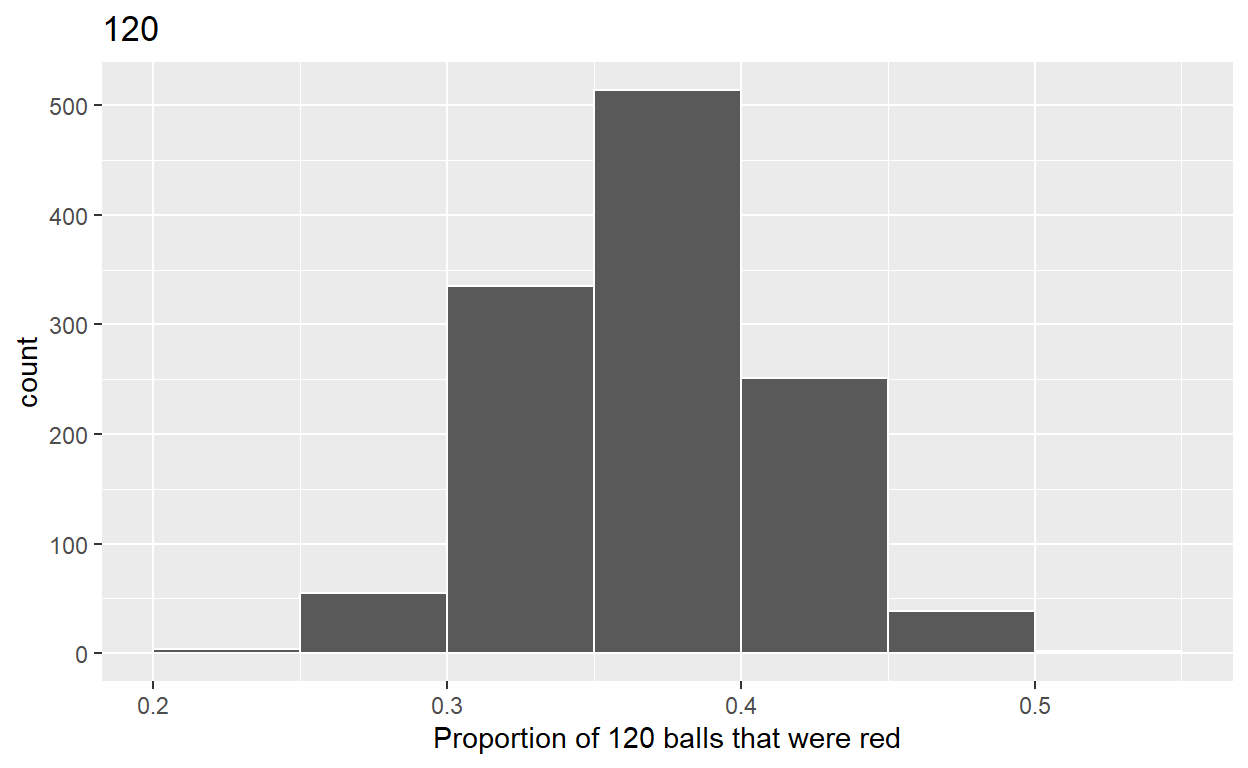# Sampling

Code for Quiz 11.

1. Load the R package we will use.
``````library(tidyverse)
library(moderndive)
``````

Question:

7.2.4 in Modern Dive with different sample sizes and representations

• Make sure you have installed and loaded the `tidyverse` and the `moderndive` packages

• Fill in the blanks

• Put the command you use in the Rchunks in your Rmd file for this quiz.

• Modify the code for comparing different sample sizes from the virtual `bowl`*

Segment 1: sample size = 30

1.a) Take 1200 sample sizes of 30 instead of 1000 replicates of size 25 from the `bowl` dataset. Assign the output to `virtual_samples_30`

``````virtual_samples_30  <- bowl  %>%
rep_sample_n(size = 30, reps = 1200)
``````

1.b) Compute resulting 1200 replicates of proportion of red - start with virtual_samples_30 THEN - group_by replicate THEN - create variable red equal to the sum of all the red balls - create variable prop_red equal to variable red/30 - Assign the output to virtual_prop_red_30

``````virtual_prop_red_30  <-  virtual_samples_30  %>%
group_by(replicate) %>%
summarize(red = sum(color == "red")) %>%
mutate(prop_red = red / 30)
``````

1.c) Plot distribution of virtual_prop_red_30 via a histogram use labs to - label x axis = “Proportion of 30 balls that were red” - create title = “30”

``````ggplot(virtual_prop_red_30, aes(x = prop_red)) +
geom_histogram(binwidth = 0.05, boundary = 0.4, color = "white")
````````````labs(x = "Proportion of 30 balls that were red", title = "30")
``````
``````\$x
 "Proportion of 30 balls that were red"

\$title
 "30"

attr(,"class")
 "labels"``````

Segment 2: sample size = 55

2.a) Take 1200 samples of size 55 instead of 1000 replicates of size 50. Assign the output to virtual_samples_55

``````virtual_samples_55  <- bowl  %>%
rep_sample_n(size = 55, reps = 1200)
``````

2.b) Compute resulting 1200 replicates of proportion red - start with virtual_samples_55 THEN - group_by replicate THEN - create variable red equal to the sum of all the red balls - create variable prop_red equal to variable red / 55 - Assign the output to virtual_prop_red_55

``````virtual_prop_red_55 <- virtual_samples_55  %>%
group_by(replicate) %>%
summarize(red = sum(color == "red")) %>%
mutate(prop_red = red / 55)
``````

2.c) Plot distribution of virtual_prop_red_55 via a histogram use labs to - label x axis = “Proportion of 55 balls that were red” - create title = “55”

``````ggplot(virtual_prop_red_55, aes(x = prop_red)) +
geom_histogram(binwidth = 0.05, boundary = 0.4, color = "white") +
labs(x = "Proportion of 55 balls that were red", title = "55")
``````Segment 3: sample size = 120

3.a) Take 1200 samples of size of 120 instead of 1000 replicates of size 50. Assign the output to virtual_samples_120

``````virtual_samples_120  <- bowl  %>%
rep_sample_n(size = 120, reps = 1200)
``````

3.b) Compute resulting 1200 replicates of proportion red - start with virtual_samples_120 THEN - group_by replicate THEN - create variable red equal to the sum of all the red balls - create variable prop_red equal to variable red / 120 - Assign the output to virtual_prop_red_120

``````virtual_prop_red_120  <- virtual_samples_120 %>%
group_by(replicate) %>%
summarize(red = sum(color == "red")) %>%
mutate(prop_red = red / 120)
``````

3.c) Plot distribution of virtual_prop_red_120 via a histogram use labs to - label x axis = “Proportion of 120 balls that were red” - create title = “120”

``````ggplot(virtual_prop_red_120, aes(x = prop_red)) +
geom_histogram(binwidth = 0.05, boundary = 0.4, color = "white") +
labs(x = "Proportion of 120 balls that were red", title = "120")
``````Calculate the standard deviations for your three sets of 1200 values of `prop_red` using the `standard deviation`

n = 30
``````virtual_prop_red_30 %>%
summarize(sd = sd(prop_red))
``````
``````# A tibble: 1 x 1
sd
<dbl>
1 0.0865``````
n = 55
``````virtual_prop_red_55 %>%
summarize(sd = sd(prop_red))
``````
``````# A tibble: 1 x 1
sd
<dbl>
1 0.0645``````
n = 120
``````virtual_prop_red_120 %>%
summarize(sd = sd(prop_red))
``````
``````# A tibble: 1 x 1
sd
<dbl>
1 0.0432``````